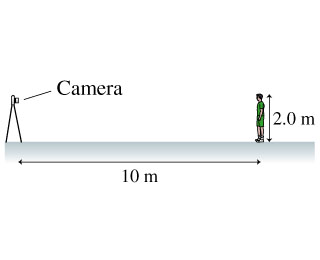# Problem: In (Figure 1) the camera lens has a 50mm focal length.How high is the man's well-focused image on the CCD detector?

###### FREE Expert Solution

Lens equation:
$\overline{)\frac{\mathbf{1}}{{\mathbit{s}}_{\mathbit{o}}}{\mathbf{+}}\frac{\mathbf{1}}{{\mathbit{s}}_{\mathbit{i}}}{\mathbf{=}}\frac{\mathbf{1}}{\mathbit{f}}}$
, where so is the object distance, si is the image distance, and f is the focal length.

Magnification:

$\overline{){\mathbf{m}}{\mathbf{=}}\frac{{\mathbf{h}}_{\mathbf{i}}}{{\mathbf{h}}_{\mathbf{o}}}{\mathbf{=}}{\mathbf{-}}\frac{{\mathbf{s}}_{\mathbf{i}}}{{\mathbf{s}}_{\mathbf{o}}}}$, where hi is the height of the image and ho is the height of the object.

93% (254 ratings)###### Problem Details

In (Figure 1) the camera lens has a 50mm focal length.How high is the man's well-focused image on the CCD detector?

Frequently Asked Questions

What scientific concept do you need to know in order to solve this problem?

Our tutors have indicated that to solve this problem you will need to apply the Thin Lens And Lens Maker Equations concept. You can view video lessons to learn Thin Lens And Lens Maker Equations. Or if you need more Thin Lens And Lens Maker Equations practice, you can also practice Thin Lens And Lens Maker Equations practice problems.